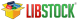`FIR Filter Library  mikroC PRO for PIC32 Libraries > DSP Libraries > ` `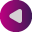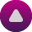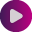`

## FIR Filter Library

mikroC PRO for PIC32 includes a library for finite impulse response (FIR) filter. All routines work with fractional Q15 format.

A finite impulse response (FIR) filter is a type of a digital filter, whose impulse response (the filter's response to a delta function) is finite because it settles to zero in a finite number of sample intervals.

### Library Routines

Prototype int FIR_Radix(const int *ptrCoeffs, unsigned FilterOrder, int *ptrInput, unsigned InputLength, unsigned Index); This function applies FIR filter to `ptrInput`. `ptrCoeffs:` pointer to filter coefficients in program memory. `FilterOrder:` order of the filter + 1. `ptrInput:` pointer to input samples. `InputLength` number of input samples. `Index:` index of current sample. Function returns value of the `Index`-th sample of the filtered signal :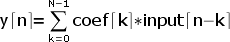with : `N` - filter order `k` - current index Nothing. ```// Filter setup: // Filter kind: FIR // Filter type: Lowpass filter // Filter order: 3 // Filter window: Blackman // Filter borders: // Wpass:4000 Hz const unsigned FILTER_ORDER = 3; const int COEFF_B[FILTER_ORDER+1] = { 0x0000, 0x1255, 0x1255, 0x0000}; int inputSamples = {0x4000, 0x2000, 0x1000}; int outputSamples; unsigned i; void main() { for (i = 0; i < 6; i++) outputSamples[i] = FIR_Radix(COEFF_B, FILTER_ORDER+1, inputSamples, 3, i); // outputSamples = COEFF_B*inputSamples; // outputSamples = COEFF_B*inputSamples + COEFF_B*inputSamples; // outputSamples = COEFF_B*inputSamples + COEFF_B*inputSamples + COEFF_B*inputSamples; // . // . // . } ``` None.
Find them on